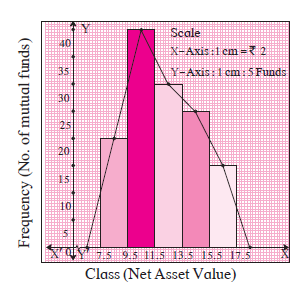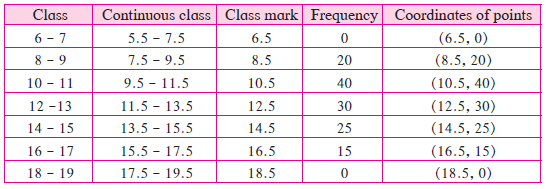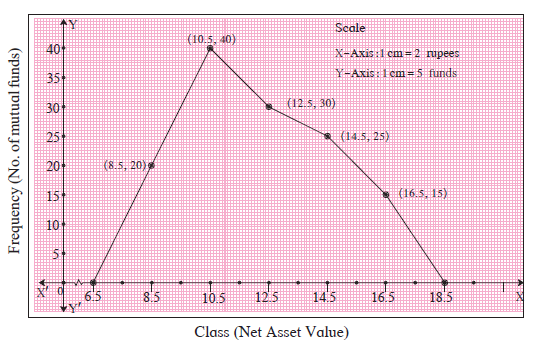# Frequency Polygon

## Notes

The information in a frequency table can be presented in various ways. We have studied a histogram. A frequency polygon is another way of presentation.
Let us study two methods of drawing a frequency polygon.
(1) With the help of a histogram (2) Without the help of a histogram.

(1) We shall use the histogram in figure  to learn the method of drawing a frequency polygon.1. Mark the mid - point of upper side of each rectangle in the histogram.
2. Assume that a rectangle of zero height exists preceeding the first rectangle and mark its mid - point. Similarly, assume a rectangle succeeding the last rectangle and mark its mid -point.
3. Join all mid - points in order by line segments.
4. The closed figure so obtained is the frequency polygon.

(2) Observe the following table. It shows how the coordinates of points are decided to draw a frequency polygon, without drawing a histogram.The points corresponding to the coordinates in the fifth column are plotted. Joining them in order by line segments, we get a frequency polygon. The polygon is shown in figure . Observe it.If you would like to contribute notes or other learning material, please submit them using the button below.